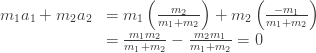This bookmarklet renders Tex/LaTeX, MathML and AsciiMathML notation on pages dynamically using the MathJax library.

### MathML Examples

#### Curl of a Vector Field

$∇→×F→=(∂Fz∂y−∂Fy∂z)i+(∂Fx∂z−∂Fz∂x)j+(∂Fy∂x−∂Fx∂y)k$

#### Definition of Christoffel Symbols

$(∇XY)k=Xi(∇iY)k=Xi(∂Yk∂xi+ΓimkYm)$

#### Standard Deviation

$σ=1N∑i=1N(xi−μ)2.$

### AsciiMathML Example

Solving the quadratic equation. Suppose a x^2+b x+c=0 and a!=0. We first divide by \a to get x^2+b/a x+c/a=0. Then we complete the square and obtain x^2+b/a x+(b/(2a))^2-(b/(2a))^2+c/a=0. The first three terms factor to give (x+b/(2a))^2=(b^2)/(4a^2)-c/a. Now we take square roots on both sides and get x+b/(2a)=+-sqrt((b^2)/(4a^2)-c/a). Finally we move the b/(2a) to the right and simplify to get the two solutions: x_(1,2)=(-b+-sqrt(b^2-4a c))/(2a)`

### WordPress.com/Wikipedia image replacement example

(Use the WordPress.com/Wikipedia bookmarklet to replace this image with MathJax)$\begin{array}{rl} m_1a_1 + m_2a_2 &= m_1 \left( \frac{m_2}{m_1+m_2} \right) + m_2 \left( \frac{-m_1}{m_1+m_2} \right) \\ &= \frac{m_1m_2}{m_1+m_2} - \frac{m_2m_1}{m_1+m_2} = 0 \end{array}$